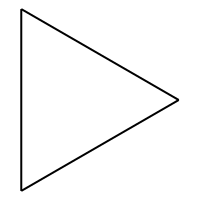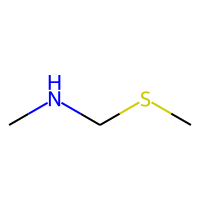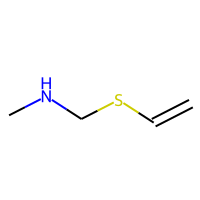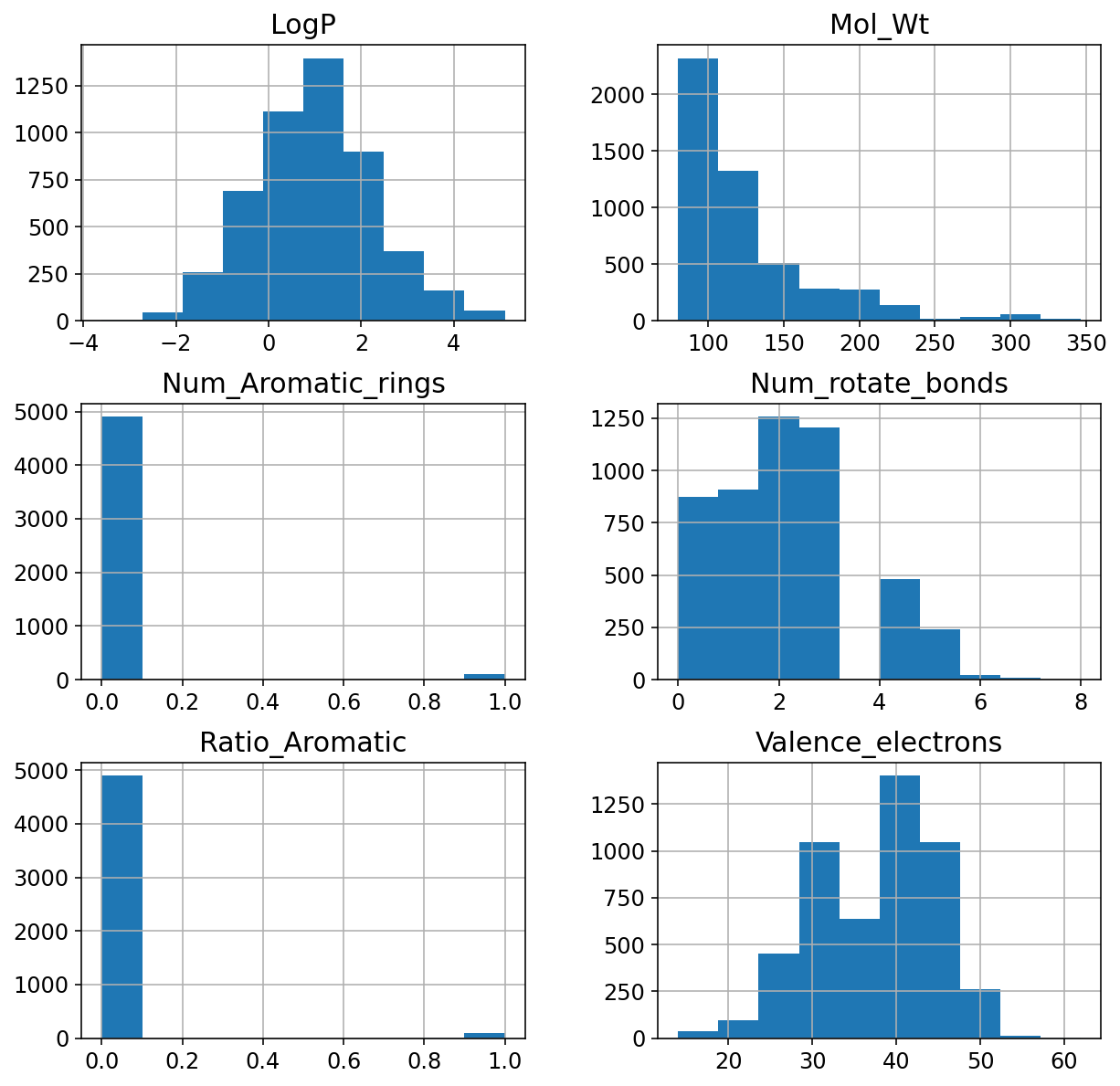Walkthrough on reading, visualizing, manipulating molecules and their properties using Pandas and RDKit for Cheminformatics-related tasks. In this file we will be primarly using SMILES to describe the molecules of choice.

### What are SMILES?

Simplified molecular input line entry system (SMILES) is a form of line notation for describing the structure of chemical species using text strings. More details can be found here

### Other resources:

1. Nice low-barrier intro to using some basic functions in RDkit: Xinhao Lin's RDKit Cheatsheet, I've adopted some of the functions frrom that blog in here:
2. Rdkit Tutorial github
3. Patrick Walters' Learning Cheminformatics Post
4. Getting Started RDKit Official Blog

Compilation of various recipes submitted by the community:

import os
import pandas as pd
import numpy as np


The majority of the basic molecular functionality is found in module rdkit.Chem

import rdkit
from rdkit import Chem #This gives us most of RDkits's functionality
from rdkit.Chem import Draw
from rdkit.Chem.Draw import IPythonConsole #Needed to show molecules
IPythonConsole.ipython_useSVG=True  #SVG's tend to look nicer than the png counterparts
print(rdkit.__version__)

# Mute all errors except critical
Chem.WrapLogs()
lg = rdkit.RDLogger.logger()
lg.setLevel(rdkit.RDLogger.CRITICAL)

2021.03.2

import matplotlib.pyplot as plt
from matplotlib.pyplot import cm

# High DPI rendering for mac
%config InlineBackend.figure_format = 'retina'

# Plot matplotlib plots with white background:
%config InlineBackend.print_figure_kwargs={'facecolor' : "w"}

plot_params = {
'font.size' : 15,
'axes.titlesize' : 15,
'axes.labelsize' : 15,
'axes.labelweight' : 'bold',
'xtick.labelsize' : 12,
'ytick.labelsize' : 12,
}

plt.rcParams.update(plot_params)


## Basics

mol = Chem.MolFromSmiles("c1ccccc1")


mol is a special type of RDkit object

type(mol)

rdkit.Chem.rdchem.Mol

To display the molecule:

mol


By default SMILES (atleast the ones we deal with RDkit) accounts H atoms connected with C atoms implicitly based on the valence of the bond. You can add H explicitly using the command below:

Chem.AddHs(mol)

Chem.RemoveHs(mol)

def mol_with_atom_index(mol):
for atom in mol.GetAtoms():
atom.SetAtomMapNum(atom.GetIdx())
return mol

mol_with_atom_index(mol)

IPythonConsole.drawOptions.addAtomIndices = True
mol = Chem.MolFromSmiles("c1ccccc1")
mol


### Descriptors for molecules

We can find more information about the molecule using rdkit.Chem.Descriptors : More information here

from rdkit.Chem import Descriptors

mol_wt = Descriptors.ExactMolWt(mol)
print('Mol Wt: {:0.3f}'.format(mol_wt))

Mol Wt: 78.047

heavy_mol_wt = Descriptors.HeavyAtomMolWt(mol)
print('Heavy Mol Wt: {:0.3f}'.format(heavy_mol_wt))

Heavy Mol Wt: 72.066

ring_count = Descriptors.RingCount(mol)
print('Number of rings: {:0.3f}'.format(ring_count))

Number of rings: 1.000

rotatable_bonds = Descriptors.NumRotatableBonds(mol)
print('Number of rotatable bonds: {:0.3f}'.format(rotatable_bonds))

Number of rotatable bonds: 0.000

num_aromatic_rings = Descriptors.NumAromaticRings(mol)
print('Number of aromatic rings: {:0.3f}'.format(num_aromatic_rings))

Number of aromatic rings: 1.000


MolToMolBlock: To get Coordinates and bonding details for the molecule

mol_block = Chem.MolToMolBlock(mol)
print(mol_block)

     RDKit          2D

6  6  0  0  0  0  0  0  0  0999 V2000
1.5000    0.0000    0.0000 C   0  0  0  0  0  0  0  0  0  0  0  0
0.7500   -1.2990    0.0000 C   0  0  0  0  0  0  0  0  0  0  0  0
-0.7500   -1.2990    0.0000 C   0  0  0  0  0  0  0  0  0  0  0  0
-1.5000    0.0000    0.0000 C   0  0  0  0  0  0  0  0  0  0  0  0
-0.7500    1.2990    0.0000 C   0  0  0  0  0  0  0  0  0  0  0  0
0.7500    1.2990    0.0000 C   0  0  0  0  0  0  0  0  0  0  0  0
1  2  2  0
2  3  1  0
3  4  2  0
4  5  1  0
5  6  2  0
6  1  1  0
M  END



## Little more on the molecule drawing

Additional discussion on different ways to represnt and draw molecules in RDkit. This section will work in RDkit version > 2020.03.

I am following the code introduced in the official RDkit blogpost

from collections import defaultdict
from rdkit.Chem.Draw import rdMolDraw2D
from IPython.display import SVG

diclofenac = Chem.MolFromSmiles('O=C(O)Cc1ccccc1Nc1c(Cl)cccc1Cl')
diclofenac


Substructure highlights: Let's look at the the C=O and the -NH species in the molecule

sub_pattern = Chem.MolFromSmarts('O=CCccN')
hit_ats = list(diclofenac.GetSubstructMatch(sub_pattern))
hit_bonds = []

for bond in sub_pattern.GetBonds():
aid1 = hit_ats[bond.GetBeginAtomIdx()]
aid2 = hit_ats[bond.GetEndAtomIdx()]

hit_bonds.append( diclofenac.GetBondBetweenAtoms(aid1, aid2).GetIdx() )

d2d = rdMolDraw2D.MolDraw2DSVG(400, 400) # or MolDraw2DCairo to get PNGs
rdMolDraw2D.PrepareAndDrawMolecule(d2d, diclofenac, highlightAtoms=hit_ats,  highlightBonds=hit_bonds)
d2d.FinishDrawing()
SVG(d2d.GetDrawingText())


Specify individual color and bonds

rings = diclofenac.GetRingInfo()
type(rings)

rdkit.Chem.rdchem.RingInfo

colors = [(0.8,0.0,0.8),(0.8,0.8,0),(0,0.8,0.8),(0,0,0.8)]

athighlights = defaultdict(list)

for i,rng in enumerate(rings.AtomRings()):
for aid in rng:
athighlights[aid].append(colors[i])

bndhighlights = defaultdict(list)
for i,rng in enumerate(rings.BondRings()):
for bid in rng:
bndhighlights[bid].append(colors[i])

d2d = rdMolDraw2D.MolDraw2DSVG(400,400)
d2d.FinishDrawing()
SVG(d2d.GetDrawingText())


## Reading dataset of molecules from a csv file

Here we will use Pandas, RDkit to make molecule object for the small sample of molecules.

## Sample data

sample_df = pd.read_csv('./data/sample_molecules.csv', sep=',')

sample_df.head(5)

Name SMILE
0 Cyclopropane C1CC1
1 Ethylene C=C
2 Methane C
3 t-Butanol CC(C)(C)O
4 ethane CC
sample_df.shape

(115, 2)
from rdkit.Chem import PandasTools


PandasTools module helps add mol molecule objects from RDKit as per the SMILES in the dataframe

PandasTools.AddMoleculeColumnToFrame(sample_df, smilesCol='SMILE')


Check the new ROMol columns being appended in the dataframe

sample_df.columns

Index(['Name', 'SMILE', 'ROMol'], dtype='object')
sample_df.head(1)

Name SMILE ROMol
0 Cyclopropane C1CC1Visualize the dataframe, add properties of interest at the bottom, you can add index too if need

PandasTools.FrameToGridImage(sample_df[:20], legendsCol='Name', molsPerRow=4)


### LogP Dataset

(From Wikipedia) The partition coefficient, abbreviated P, is defined as the ratio of the concentrations of a solute between two immisible solvents at equilibrium. Most commonly, one of the solvents is water, while the second is hydrophobic, such as 1-octanol.

$$\log P_\text{oct/wat} = \log\left(\frac{\big[\text{solute}\big]_\text{octanol}^\text{un-ionized}}{\big[\text{solute}\big]_\text{water}^\text{un-ionized}}\right)$$

Hence the partition coefficient measures how hydrophilic ("water-loving") or hydrophobic ("water-fearing") a chemical substance is. Partition coefficients are useful in estimating the distribution of drugs within the body. Hydrophobic drugs with high octanol-water partition coefficients are mainly distributed to hydrophobic areas such as lipid bilayers of cells. Conversely, hydrophilic drugs (low octanol/water partition coefficients) are found primarily in aqueous regions such as blood serum.

The dataset used in this notebook is obtained from Kaggle. This dataset features relatively simple molecules along with their LogP value. This is a synthetic dataset created using XLogP and does not contain experimental validation.

logP_df = pd.read_csv('./data/logP_dataset.csv', sep=',', header=None, names=['SMILES', 'LogP'])

logP_df.head(5)

SMILES LogP
0 C[C@H]([C@@H](C)Cl)Cl 2.3
1 C(C=CBr)N 0.3
2 CCC(CO)Br 1.3
3 [13CH3][13CH2][13CH2][13CH2][13CH2][13CH2]O 2.0
4 CCCOCCP 0.6
logP_df.shape

(14610, 2)

### Visualize the SMILE string

mol_temp = logP_df.iloc

mol_temp

SMILES    [2H][C]([2H])[Cl+]Cl
LogP                       1.6
Name: 420, dtype: object
mol_obj = Chem.MolFromSmiles(mol_temp['SMILES'])
mol_obj

print(Chem.MolToMolBlock(mol_obj))

     RDKit          2D

5  4  0  0  0  0  0  0  0  0999 V2000
1.2990    0.7500    0.0000 H   0  0  0  0  0  0  0  0  0  0  0  0
0.0000    0.0000    0.0000 C   0  0  0  0  0  3  0  0  0  0  0  0
-1.2990    0.7500    0.0000 H   0  0  0  0  0  0  0  0  0  0  0  0
-0.0000   -1.5000    0.0000 Cl  0  0  0  0  0  2  0  0  0  0  0  0
-1.2990   -2.2500    0.0000 Cl  0  0  0  0  0  0  0  0  0  0  0  0
1  2  1  0
2  3  1  0
2  4  1  0
4  5  1  0
M  CHG  1   4   1
M  ISO  2   1   2   3   2
M  END



Take a small sample from QM9 dataset

logP_df_smol = logP_df.sample(20).reset_index(drop=True)

logP_df_smol.head(2)

SMILES LogP
0 CCCSSCC 2.2
1 C(CO)C(N)Br -0.1
logP_df_smol.shape

(20, 2)

PandasTools module helps add mol molecule objects from RDKit as per the SMILES in the dataframe

PandasTools.AddMoleculeColumnToFrame(logP_df_smol, smilesCol='SMILES')


Check the new ROMol columns being appended in the dataframe

logP_df_smol.columns

Index(['SMILES', 'LogP', 'ROMol'], dtype='object')
logP_df_smol['ROMol']


Visualize the dataframe, add properties of interest at the bottom, you can add index too if need

PandasTools.FrameToGridImage(logP_df_smol, legendsCol='LogP', molsPerRow=3, subImgSize=(200,200))


## Vanilla linear regression

Let's try building a model to predict a molecule's logP value given other descriptors. We will try simple molecular descriptors and check the performance. Some molecule discriptors we will consider:

1. Molecular weight
2. Number of rotatable bonds
3. Number of aromatic compounds
logP_df.head(4)

SMILES LogP
0 C[C@H]([C@@H](C)Cl)Cl 2.3
1 C(C=CBr)N 0.3
2 CCC(CO)Br 1.3
3 [13CH3][13CH2][13CH2][13CH2][13CH2][13CH2]O 2.0

As before we will first convert the SMILES string into a rdkit.Chem.rdchem.Mol object, let's write a convenience function to do so

_count = 0
for i in range(mol.GetNumAtoms()):
if mol.GetAtomWithIdx(i).GetIsAromatic():
_count = _count + 1
print(_count)

6

def generate_variables(smiles_list):

variable_array = {'SMILES':[], 'ROMol':[], 'Mol_Wt':[],'Num_Aromatic_rings':[], 'Num_rotate_bonds':[], 'Ratio_Aromatic':[], 'Valence_electrons':[]}

for smile_entry in smiles_list:
mol_object = Chem.MolFromSmiles(smile_entry)

mol_wt = Descriptors.MolWt(mol_object)
mol_aromatic_rings = Descriptors.NumAromaticRings(mol_object)
mol_rotatable_bonds = Descriptors.NumRotatableBonds(mol_object)

# Calculate % of aromatic atoms in the compd
mol_num_heavy_atom = Descriptors.HeavyAtomCount(mol_object)

_count_aromatic = 0
for i in range(mol_object.GetNumAtoms()):
if mol_object.GetAtomWithIdx(i).GetIsAromatic() == True:
_count_aromatic = _count_aromatic + 1

mol_aromatic_ratio = _count_aromatic / mol_num_heavy_atom

mol_val_electrons = Descriptors.NumValenceElectrons(mol_object)

variable_array['SMILES'].append(smile_entry)
variable_array['ROMol'].append(mol_object)
variable_array['Mol_Wt'].append(mol_wt)
variable_array['Num_Aromatic_rings'].append(mol_aromatic_rings)
variable_array['Num_rotate_bonds'].append(mol_rotatable_bonds)
variable_array['Ratio_Aromatic'].append(mol_aromatic_ratio)
variable_array['Valence_electrons'].append(mol_val_electrons)

return variable_array


### Look at a subset from the total logP data

df_5k = logP_df.sample(5000, random_state=42)

variable_dict = generate_variables(df_5k.SMILES)
variable_df = pd.DataFrame(variable_dict, columns=variable_dict.keys())

df_var_5k = df_5k.merge(variable_df, on='SMILES')

SMILES LogP ROMol Mol_Wt Num_Aromatic_rings Num_rotate_bonds Ratio_Aromatic Valence_electrons
0 CNCSC 0.591.179 0 2 0.0 32
1 CNCSC=C 1.0103.190 0 3 0.0 36

### Setup model

df_var_5k.columns

Index(['SMILES', 'LogP', 'ROMol', 'Mol_Wt', 'Num_Aromatic_rings',
'Num_rotate_bonds', 'Ratio_Aromatic', 'Valence_electrons'],
dtype='object')
fig, ax = plt.subplots(1,1, figsize=(10,10))
df_var_5k.hist(ax = ax);

<ipython-input-48-9e19fbae4460>:2: UserWarning: To output multiple subplots, the figure containing the passed axes is being cleared
df_var_5k.hist(ax = ax);Split into train and validation set

from sklearn.preprocessing import StandardScaler
from sklearn.model_selection import train_test_split

df_train, df_test = train_test_split(df_var_5k, test_size=0.3, random_state=42)

print(df_train.shape, df_test.shape)

(3500, 8) (1500, 8)


Drop some columns that arent that useful for prediction

X_train = df_train.drop(columns = ['LogP','ROMol','SMILES', 'Ratio_Aromatic', 'Num_Aromatic_rings']).values
y_train = df_train.LogP.values


Pre-process input data to normalize the scale of the descriptors

std_scaler = StandardScaler()
X_train_std = std_scaler.fit_transform(X_train)

from sklearn.linear_model import LinearRegression

model = LinearRegression()
model.fit(X_train_std, y_train)

LinearRegression()
y_pred = model.predict(X_train_std)

plt.scatter(y_train, y_pred, alpha=0.6)
plt.xlabel('True Value')
plt.ylabel('Predicted')

Text(0, 0.5, 'Predicted')fig, ax = plt.subplots(1,1, figsize=(5,5))
ax.scatter(y_train, y_pred, alpha=0.6, label='Linear Regression')

lims = [np.min([ax.get_xlim(), ax.get_ylim()]),  # min of both axes
np.max([ax.get_xlim(), ax.get_ylim()]),  # max of both axes
]
# Linear fit
reg = np.polyfit(y_train, y_pred, deg=1)
ax.plot(lims, reg * np.array(lims) + reg, 'r--', linewidth=1.5, label='Linear Fit')
ax.plot(lims, lims, 'k--', alpha=0.75, zorder=0, label='Parity Line')
ax.set_aspect('equal')

ax.set_xlabel('True value')
ax.set_ylabel('Model predicted')
ax.legend(loc='best');